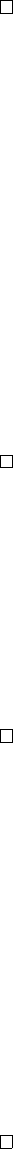8Matrix groups

IA Groups8.3 Orthogonal groups
Recall that
A
T
is defined by
A
T
ij
=
A
ji
, i.e. we reflect the matrix in the diagonal.
They have the following properties:
(i) (AB)
T
= B
T
A
T
(ii) (A
1
)
T
= (A
T
)
1
(iii) A
T
A = I AA
T
= I A
1
= A
T
. In this case A is orthogonal
(iv) det A
T
= det A
We are now in
R
, because orthogonal matrices don’t make sense with complex
matrices.
Note that a matrix is orthogonal if the columns (or rows) form an orthonormal
basis of
R
n
:
AA
T
=
I a
ik
a
jk
=
δ
ij
a
i
· a
j
=
δ
ij
, where
a
i
is the
i
th column
of A.
The importance of orthogonal matrices is that they are the isometries of
R
n
.
Lemma
(Orthogonal matrices are isometries)
.
For any orthogonal
A
and
x, y
R
n
, we have
(i) (Ax) · (Ay) = x · y
(ii) |Ax| = |x|
Proof. Treat the dot product as a matrix multiplication. So
(Ax)
T
(Ay) = x
T
A
T
Ay = x
T
Iy = x
T
y
Then we have
|Ax|
2
= (
Ax
)
·
(
Ax
) =
x · x
=
|x|
2
. Since both are positive, we
know that |Ax| = |x|.
It is important to note that orthogonal matrices are isometries, but not all
isometries are orthogonal. For example, translations are isometries but are not
represented by orthogonal matrices, since they are not linear maps and cannot
be represented by matrices at all! However, it is true that all linear isometries
can be represented by orthogonal matrices.
Definition (Orthogonal group O(n)). The orthogonal group is
O(n) = O
n
= O
n
(R) = {A GL
n
(R) : A
T
A = I},
i.e. the group of orthogonal matrices.
We will later show that this is the set of matrices that preserve distances in
R
n
.
Lemma. The orthogonal group is a group.
Proof.
We have to check that it is a subgroup of
GL
n
(
R
): It is non-empty,
since
I
O(
n
). If
A, B
O(
n
), then (
AB
1
)(
AB
1
)
T
=
AB
1
(
B
1
)
T
A
T
=
AB
1
BA
1
= I, so AB
1
O(n) and this is indeed a subgroup.
Proposition. det : O(n) 1} is a surjective group homomorphism.
Proof.
For
A
O(
n
), we know that
A
T
A
=
I
. So
det A
T
A
= (
det A
)
2
= 1. So
det A = ±1. Since det(AB) = det A det B, it is a homomorphism. We have
det I = 1, det
1 0 · · · 0
0 1 · · · 0
.
.
.
.
.
.
.
.
.
0
0 0 · · · 1
= 1,
so it is surjective.
Definition
(Special orthogonal group
SO
(
n
))
.
The special orthogonal group is
the kernel of det : O(n) 1}.
SO(n) = SO
n
= SO
n
(R) = {A O(n) : det A = 1}.
By the isomorphism theorem, O(n)/SO(n)
=
C
2
.
What’s wrong with matrices with determinant
1? Why do we want to
eliminate these? An important example of an orthogonal matrix with determinant
1 is a reflection. These transformations reverse orientation, and is often
unwanted.
Lemma. O(n) = SO(n)
1 0 · · · 0
0 1 · · · 0
.
.
.
.
.
.
.
.
.
0
0 0 · · · 1
SO(n)
Proof. Cosets partition the group.